Ask question

# Consider the non-triangle shown below that has side lenghts of 1.6, 1.751 and 2.6 cm and interior angle measures of 0.72, alpha and beta degrees.What is value of alpha and beta01510101641.png# Consider the non-triangle shown below that has side lenghts of 1.6, 1.751 and 2.6 cm and interior angle measures of 0.72, alpha and beta degrees.What is value of alpha and beta01510101641.png

Question
Non-right triangles and trigonometryasked 2021-01-31

Consider the non-triangle shown below that has side lenghts of 1.6, 1.751 and 2.6 cm and interior angle measures of 0.72, alpha and beta degrees.
What is value of alpha and beta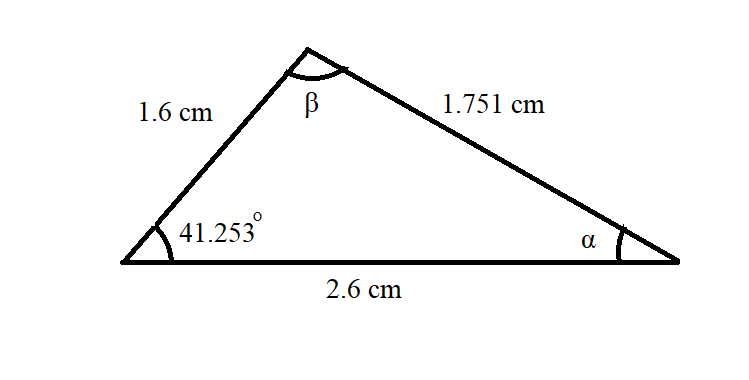## Answers (1)2021-02-01
According to sine law:
$$\displaystyle\frac{{{\sin{{A}}}}}{{a}}=\frac{{{\sin{{B}}}}}{{b}}=\frac{{{\sin{{C}}}}}{{c}}$$
Thus, (sin alpha)/1.6=(sin 41.253^@)/1.751ZSK
$$\displaystyle{\sin{\alpha}}=\frac{{{1.6}\cdot{\sin{{41.253}}}^{\circ}}}{{1.751}}$$
$$\displaystyle{\sin{\alpha}}={0.60252}$$
$$\displaystyle\therefore\alpha={37.051}^{\circ}$$
Now, using triangle property
$$\displaystyle{A}+{B}+{C}={180}^{\circ}$$
$$\displaystyle\alpha+\beta+{41.253}={180}^{\circ}$$
$$\displaystyle{37.051}+\beta={180}^{\circ}-{41.253}^{\circ}$$
$$\displaystyle\beta={138.747}-{37.051}$$
$$\displaystyle\therefore\beta={101.696}^{\circ}$$
Therefore,
$$\displaystyle\alpha={37.051}^{\circ}$$
$$\displaystyle\beta={101.696}^{\circ}$$

### Relevant Questionsasked 2021-03-05

Find the perimeter of the triangle.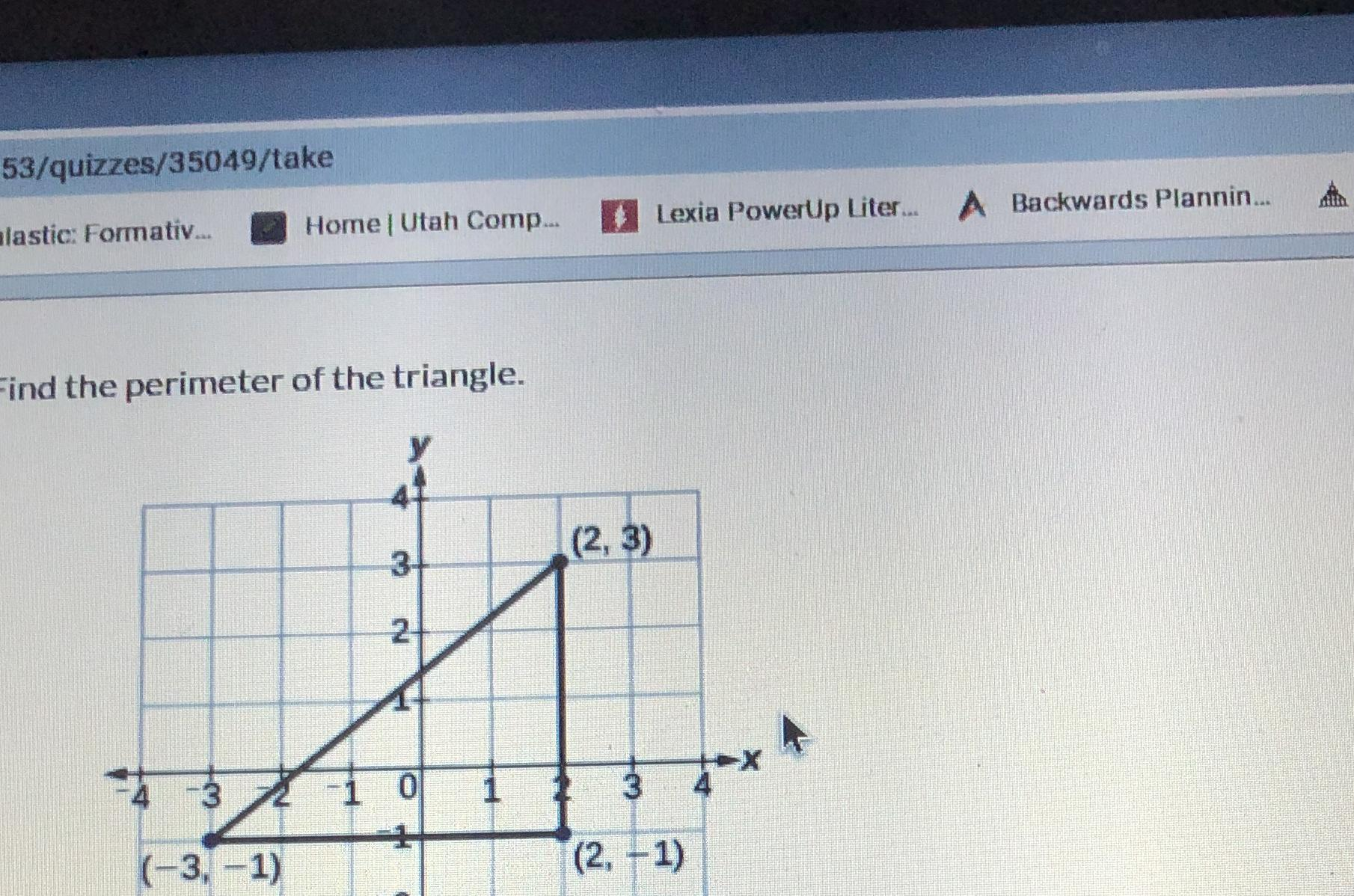asked 2021-01-04

Find the remaining angles for $$\displaystyle△{A}{B}{C}$$. $$\displaystyle{m}∠{B}={30}∘{\quad\text{and}\quad}{m}∠{A}=\frac{{1}}{{2}}{m}∠{C}$$
$$\displaystyle{m}∠{A}=√∘$$ and $$\displaystyle{m}∠{C}=√∘​$$asked 2021-01-25

In $$\triangle PQR$$, identify the hypotenuse, adjacent leg, and opposite leg for $$\angle R$$.asked 2021-03-29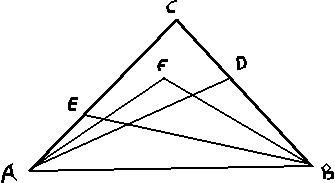In any triangle ABC, E and D are interior points of AC and BC,respectively. AF bisects angle CAD and BF bisects angle CBE. Prove that measures (AEB) + measure (ADB) = 2x measure of (AFB).asked 2020-11-26

Calculate the tension $$\displaystyle{F}_{{T}}$$ in the wire that supportsthe 30 kg beam shown in the picture, and the force $$\displaystyle{F}_{{W}}$$ exerted by the wall on the beam (give magnitude and direction.)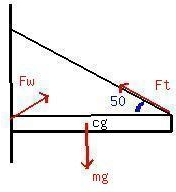asked 2021-05-05
A golf ball lies 2.00 m directly south of the hole on a levelgreen. On the first putt, the ball travels 3.00 m along astraight-line path at an angle of 5 degrees east of north; on thesecond putt, it travels a straight-line distance of 1.20 m at anangle of 6 degrees south of west.
What would be the displacement ofa third putt that would put the ball in the hole?
The answer in the back of the book is 1.3 m at 43 degreessouth of east. How do I get that?asked 2021-05-18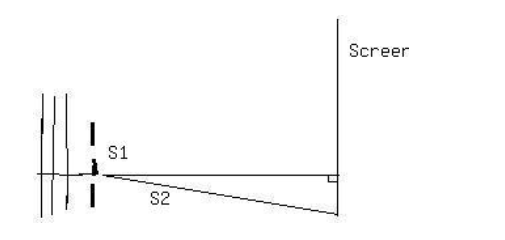1. S1 and S2, shown above, are thin parallel slits in an opaqueplate. A plane wave of wavelength λ is incident from the leftmoving in a direction perpendicular to the plate. On a screenfar from the slits there are maximums and minimums in intensity atvarious angles measured from the center line. As the angle isincreased from zero, the first minimum occurs at 3 degrees. Thenext minimum occurs at an angle of-
A. 4.5 degrees
B. 6 degrees
C. 7.5 degrees
D. 9 degrees
E. 12 degreesasked 2021-04-14
A medical technician is trying to determine what percentage of apatient's artery is blocked by plaque. To do this, she measures theblood pressure just before the region of blockage and finds that itis $$\displaystyle{1.20}\times{10}^{{{4}}}{P}{a}$$, while in the region of blockage it is $$\displaystyle{1.15}\times{10}^{{{4}}}{P}{a}$$. Furthermore, she knows that blood flowingthrough the normal artery just before the point of blockage istraveling at 30.0 cm/s, and the specific gravity of this patient'sblood is 1.06. What percentage of the cross-sectional area of thepatient's artery is blocked by the plaque?asked 2021-03-24
A man with mass 70.0 kg stands on a platform withmass 25.0 kg. He pulls on the free end of a rope thatruns over a pulley on the ceiling and has its other end fastened tothe platform. The mass of the rope and the mass of the pulley canbe neglected, and the pulley is frictionless. The rope is verticalon either side of the pulley.
a) With what force does he have to pull so that he and theplatform have an upward acceleration of $$\displaystyle{1.80}\frac{{m}}{{s}^{{2}}}$$?
b) What is the acceleration of the rope relative to him?asked 2021-05-16
Look Out! A snowball rolls off a barn roof that slopes downward at an angle of 40 degrees . The edge of the roof is 14.0 m above the ground, and the snowball has a speed of 7.00 m/s as it rolls off the roof. Ignore air resistance.
A man 1.9 m tall is standing 4.0 m from the edge of the barn. Will he be hit by the snowball?
...